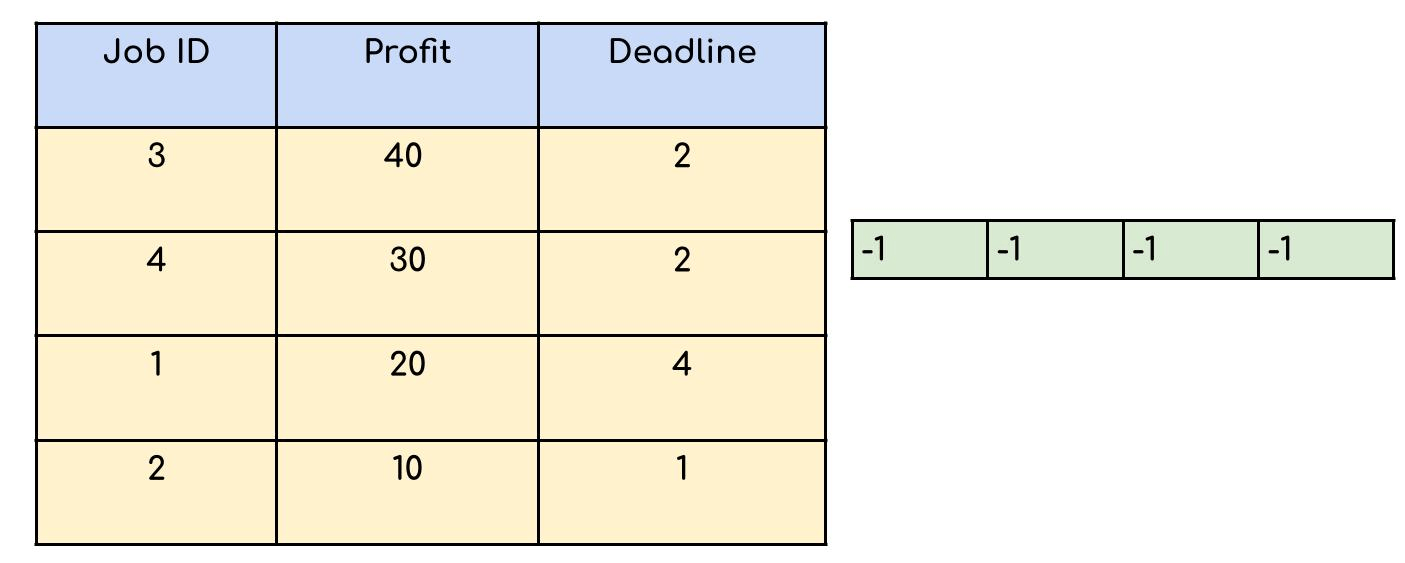# Job Sequencing Problem

Problem Statement: You are given a set of N jobs where each job comes with a deadline and profit. The profit can only be earned upon completing the job within its deadline. Find the number of jobs done and the maximum profit that can be obtained. Each job takes a single unit of time and only one job can be performed at a time.

Examples

```Example 1:

Input: N = 4, Jobs = {(1,4,20),(2,1,10),(3,1,40),(4,1,30)}

Output: 2 60

Explanation: The 3rd job with a deadline 1 is performed during the first unit of time .The 1st job is performed during the second unit of time as its deadline is 4.
Profit = 40 + 20 = 60

Example 2:

Input: N = 5, Jobs = {(1,2,100),(2,1,19),(3,2,27),(4,1,25),(5,1,15)}

Output: 2 127

Explanation: The  first and third job both having a deadline 2 give the highest profit.
Profit = 100 + 27 = 127
```

### Solution

Disclaimer: Don’t jump directly to the solution, try it out yourself first.

Approach:  The strategy to maximize profit should be to pick up jobs that offer higher profits. Hence we should sort the jobs in descending order of profit. Now say if a job has a deadline of 4 we can perform it anytime between day 1-4, but it is preferable to perform the job on its last day. This leaves enough empty slots on the previous days to perform other jobs.

Basic Outline of the approach:-

• Sort the jobs in descending order of profit.
• If the maximum deadline is x, make an array of size x .Each array index is set to -1 initially as no jobs have been performed yet.
• For every job check if it can be performed on its last day.
• If possible mark that index with the job id and add the profit to our answer.
• If not possible, loop through the previous indexes until an empty slot is found.

DRY RUN:Profit = 90 , No of jobs done = 3

Code:

## C++ Code

``````#include<bits/stdc++.h>

using namespace std;
// A structure to represent a job
struct Job {
int id; // Job Id
int profit; // Profit if job is over before or on deadline
};
class Solution {
public:
bool static comparison(Job a, Job b) {
return (a.profit > b.profit);
}
//Function to find the maximum profit and the number of jobs done
pair < int, int > JobScheduling(Job arr[], int n) {

sort(arr, arr + n, comparison);
int maxi = arr.dead;
for (int i = 1; i < n; i++) {
maxi = max(maxi, arr[i].dead);
}

int slot[maxi + 1];

for (int i = 0; i <= maxi; i++)
slot[i] = -1;

int countJobs = 0, jobProfit = 0;

for (int i = 0; i < n; i++) {
for (int j = arr[i].dead; j > 0; j--) {
if (slot[j] == -1) {
slot[j] = i;
countJobs++;
jobProfit += arr[i].profit;
break;
}
}
}

return make_pair(countJobs, jobProfit);
}
};
int main() {
int n = 4;
Job arr[n] = {{1,4,20},{2,1,10},{3,2,40},{4,2,30}};

Solution ob;
//function call
pair < int, int > ans = ob.JobScheduling(arr, n);
cout << ans.first << " " << ans.second << endl;

return 0;
}
``````

Output: 3 90

Time Complexity: O(N log N) + O(N*M).

O(N log N ) for sorting the jobs in decreasing order of profit. O(N*M) since we are iterating through all N jobs and for every job we are checking from the last deadline, say M deadlines in the worst case.

Space Complexity: O(M) for an array that keeps track on which day which job is performed if M is the maximum deadline available.

## Java Code

``````import java.io.*;
import java.lang.*;
import java.util.*;

class Job {
int id, profit, deadline;
Job(int x, int y, int z) {
this.id = x;
this.profit = z;
}
}

class solve {
// return an array of size 2 having the 0th element equal to the count
// and 1st element equal to the maximum profit
int[] JobScheduling(Job arr[], int n) {
Arrays.sort(arr, (a, b) -> (b.profit - a.profit));

int maxi = 0;
for (int i = 0; i < n; i++) {
if (arr[i].deadline > maxi) {
}
}

int result[] = new int[maxi + 1];

for (int i = 1; i <= maxi; i++) {
result[i] = -1;
}

int countJobs = 0, jobProfit = 0;

for (int i = 0; i < n; i++) {

for (int j = arr[i].deadline; j > 0; j--) {

// Free slot found
if (result[j] == -1) {
result[j] = i;
countJobs++;
jobProfit += arr[i].profit;
break;
}
}
}

int ans[] = new int;
ans = countJobs;
ans = jobProfit;
return ans;

}
}
class Main {
public static void main(String[] args) throws IOException {

//size of array
Job[] arr = new Job;
arr = new Job(1, 4, 20);
arr = new Job(2, 1, 10);
arr = new Job(3, 2, 40);
arr = new Job(4, 2, 30);

solve ob = new solve();

//function call
int[] res = ob.JobScheduling(arr, 4);
System.out.println(res + " " + res);

}
}
``````

Output: 3 90

Time Complexity: O(N log N) + O(N*M).

O(N log N ) for sorting the jobs in decreasing order of profit. O(N*M) since we are iterating through all N jobs and for every job we are checking from the last deadline, say M deadlines in the worst case.

Space Complexity: O(M) for an array that keeps track on which day which job is performed if M is the maximum deadline available.

Special thanks to Somparna Chakrabarti for contributing to this article on takeUforward. If you also wish to share your knowledge with the takeUforward fam, please check out this article